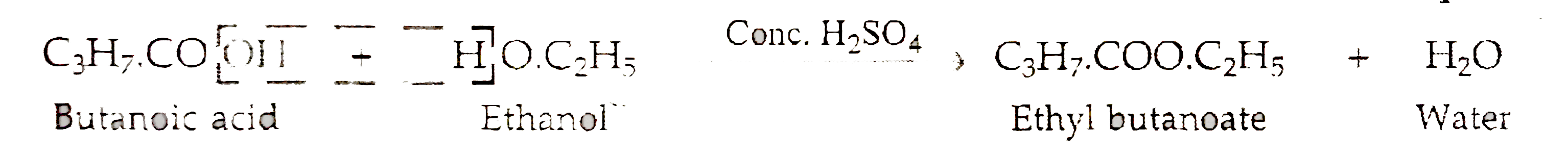# The molecular formula of an ester is C_(3)H_(7)COOC_(2)H_(5). Write the molecular formula of the alcohol and the acid from which it might be prepare

119 views

closed
The molecular formula of an ester is C_(3)H_(7)COOC_(2)H_(5). Write the molecular formula of the alcohol and the acid from which it might be prepared

by (72.0k points)
selected

The left side part of the formula of ana ester (containing the CO part ) is derived from the acid and the right side part of and easter is derived from the alcohol .So in the ester C_(3)H_(7)COOC_(2)H_(5), the acid will be C_(3)H_(7)COOH and the alcohol will be HOC_(2)H_(5) which is written more conveniently as C_(2)H_(5)OH Thus
(i) The acid present in the ester C_(3)H_(7)COOC_(2)H_(5) "is" C_(3)H_(7)COOH which is butanoic acid .
(ii) The reaction of butanoic aid and ethanol to from this ester can be represented as: